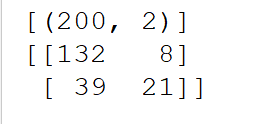python深度学习分类后的 混淆矩阵的意义``````import numpy as np
import urllib.request
import pandas as pd
from pandas import DataFrame
import numpy as np
import pandas as pd
import xlrd
from sklearn import preprocessing
def excel_to_matrix(path):
table = xlrd.open_workbook(path).sheets()  # 获取第一个sheet表
row = table.nrows  # 行数
col = table.ncols  # 列数
datamatrix = np.zeros((row, col))
for x in range(col):
cols = np.matrix(table.col_values(x))

datamatrix[:, x] = cols
return datamatrix

datafile = u'C:\\Users\\asus\\PycharmProjects\\2\\venv\\Lib\\附件2：数据.xls'
datamatrix=excel_to_matrix(datafile)
data=pd.DataFrame(datamatrix)

y=data
data=data.drop(10,1)
x=data

from sklearn import preprocessing
x_MinMax=preprocessing.MinMaxScaler()

y=np.array(y).reshape((len(y),1))

x=x_MinMax.fit_transform(x)
x.mean(axis=0)
import random
from sklearn.cross_validation import train_test_split
np.random.seed(2016)
x_train,x_test,y_train,y_test=train_test_split(x,y,test_size=0.2)

from sknn.mlp import Classifier,Layer #预测模型

fit3=Classifier(layers=[Layer('Tanh',units=38),Layer('Tanh',units=45),
Layer('Tanh', units=28),
Layer('Softmax')],
learning_rate=0.02,
random_state=2016,
n_iter=100,
dropout_rate=0.05,
batch_size=50,
learning_momentum=0.005

)
fit3.fit(x_train,y_train)

from sklearn.metrics import confusion_matrix
predict3_train=fit3.predict(x_train)
print(predict3_train)

predict3_test=fit3.predict(x_test)
confu3_test=confusion_matrix(y_test,predict3_test)
print(confu3_test)
``````

1个回答